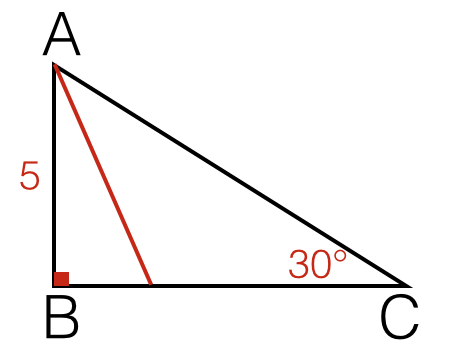# Can You Solve This Without Trigonometry?

Geometry Level 1Let $ABC$ be a triangle with $\angle B=90^\circ$, $\angle C=30^\circ$ and $AB=5$ Find the length of the angle bisector from $A$ to $\overline{BC}$(right to $2$ decimal places).

×